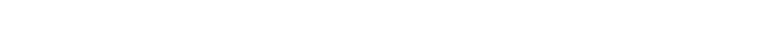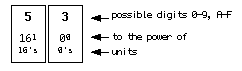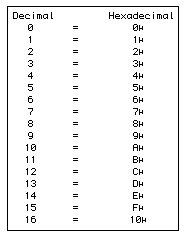# Computer counting systems by Matt OttewillDigital systems (such as computers, mobile phones, DVD players etc) use a digital (binary) code of 1s and 0s to represent information. You can read more about the physical forms binary takes here. This page explains how binary bits (1s and 0s) are combined to create larger and more meaningful units which in turn represent information and data.

#Denary / decimal (base 10)

This is our everyday counting system. Here is an example of a decimal number:

Before decimalisation in the UK we used base 12 (imperial) derived from the seasons and the phases of the moon.

#Binary (base 2)

Base 2 uses only 0’s and 1's. Computers use this. Early computers employed simple relays (electro magnets closed and released/opened) to represent 0's and 1's. Then came vacuum tubes followed by transistors. Binary is the obvious counting base for computers.

Early computers had an 8-bit architecture. This means they were able to calculate one 8 digit number at a time. These 8 digit numbers are called BYTEs. Each individual digit of a BYTE is called a BIT. 8 bit numbers can be divided into two 4 bit parts called NIBBLEs. Here is an example of an 8-bit byte. The number represented is 218:

The smallest possible number an 8-bit byte can represent = 00000000 (8 zero’s) or 0 in decimal.

The biggest number an 8-bit byte can represent = 11111111 (8 one’s) or 255 in decimal.

A NIBBLE can represent 16 numbers from 0-15. Incidentally, 1024 Bytes = 1 kilobyte (1K). 1024K (roughly a million bytes) = 1 megabyte (1mb).

MIDI sequencer users (for example) do not interface with computers at binary level. When they change value parameters in Logic or Cubase, they will be using a decimal interface. If you think about it, a sequencing program is nothing more than a piece of code which can record manipulate, replay and store MIDI events whilst presenting them to the end user graphically and numerically in a familiar counting base (ie decimal). "Under the hood" its all binary (1s and 0s).

Because MIDI is tied to a hardware specification (ports, keyboards etc) it has remained an 8-bit language. Other software elements in a computer system may be 16, 24, 32 or 64-bit. Click here to read more.

# MIDI & Hexadecimal (base 16)

Because MIDI is an 8-bit language (nice and simple to understand!) its interesting to understand how it can be expressed in base 16 (Hex), in addition to decimal and base 2. In fact MIDI is often displayed in Hex to make editing and manipulation of Systems Exclusive data easier.

Between the decimal interface of a sequencer and the underlying binary computer machine code, MIDI can be manipulated with the more succinct and convenient counting base of hexadecimal (or hex). To facilitate the control of systems exclusive messages sequencers often have screens/pages where MIDI data is presented in hexadecimal form.This is an example of a hex number:

The left hand column is the sixteen's column and the right hand column is the ones. Straight away we are faced with a problem. In the right hand column we need to be able to express in a single digit the range of numbers from 0 to 15. The solution is to use letters to express numbers above 9. Here is a table showing decimal to hex conversion:Hex numbers are followed by an H to help identify them (eg 53H). Every 8-bit MIDI value can be expressed by a 2 digit hex number. For example; the MIDI byte 10010011 is 147 in decimal or 93H in hex.

The biggest number hex can express in 2 columns (or bits) = FFH (15 x 16 + 15) = 255 in decimal.

Biggest number in 8 bit binary = 11111111 = 255.

Thus a 2 bit hex number can represent any 8 bit binary byte.

For the most part we will not need to program with hex unless we need to send specific messages not available to us as standard features of the software sequencers we use, or create MIDI Maps in Cubase, or write MIDI programs.